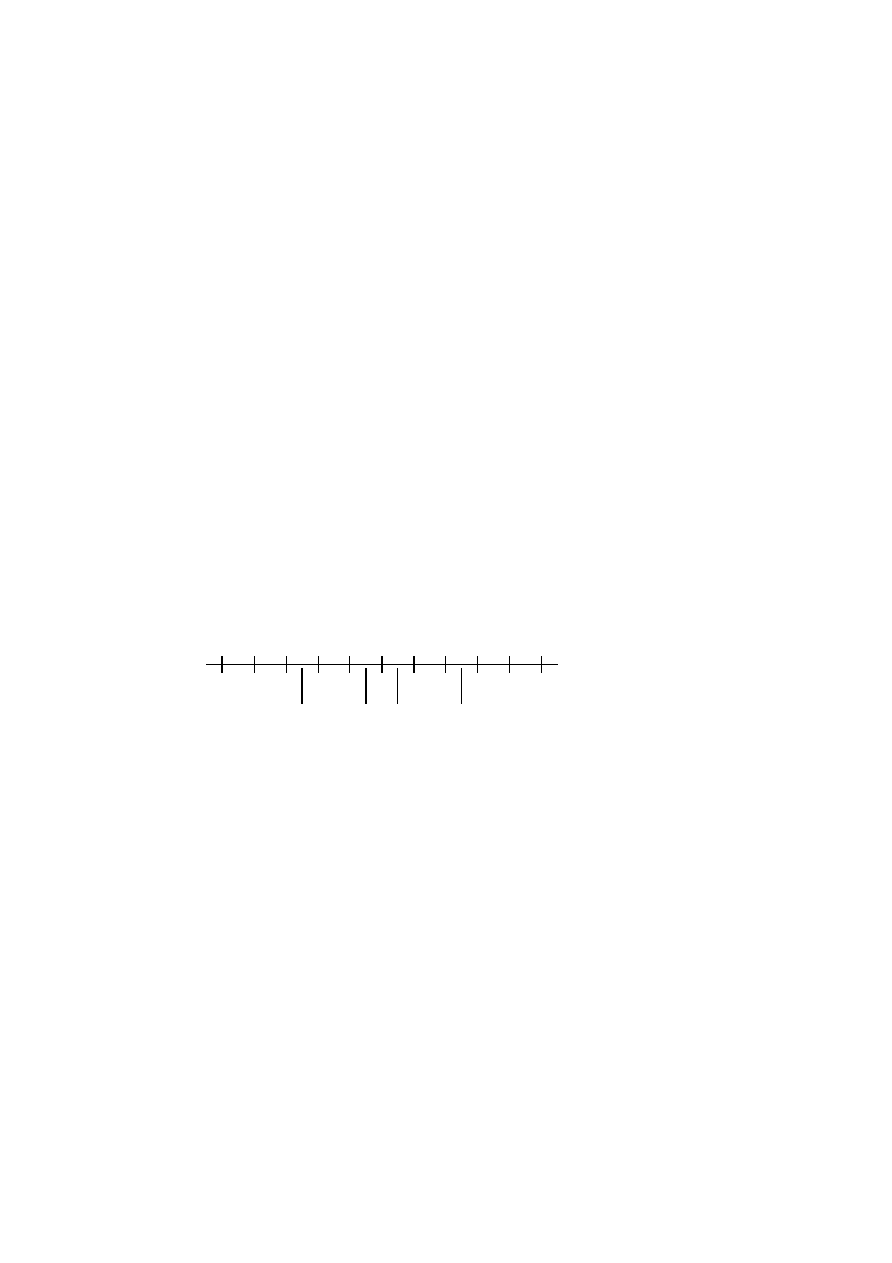ISO/IEC 10918-1 : 1993(E)
F.1.4.4
Statistical models
An adaptive binary arithmetic coder requires a statistical model. The statistical model defines the contexts which are used
to select the conditional probability estimates used in the encoding and decoding procedures.
Each decision in the binary decision trees is associated with one or more contexts. These contexts identify the sense of the
MPS and the index in Table D.3 of the conditional probability estimate Qe which is used to encode and decode the binary
decision.
The arithmetic coder is adaptive, which means that the probability estimates for each context are developed and
maintained by the arithmetic coding system on the basis of prior coding decisions for that context.
F.1.4.4.1
Statistical model for coding DC prediction differences
The statistical model for coding the DC difference conditions some of the probability estimates for the binary decisions on
previous DC coding decisions.
F.1.4.4.1.1
Statistical conditioning on sign
In coding the DC coefficients, four separate statistics bins (probability estimates) are used in coding the zero/not-zero (V
=
0) decision, the sign decision and the first magnitude category decision. Two of these bins are used to code the V
=
0
decision and the sign decision. The other two bins are used in coding the first magnitude decision, Sz < 1; one of these
bins is used when the sign is positive, and the other is used when the sign is negative. Thus, the first magnitude decision
probability estimate is conditioned on the sign of V.
F.1.4.4.1.2
Statistical conditioning on DC difference in previous block
The probability estimates for these first three decisions are also conditioned on Da, the difference value coded for the
previous DCT block of the same component. The differences are classified into five groups: zero, small positive, small
negative, large positive and large negative. The relationship between the default classification and the quantization scale is
shown in Figure F.10.
­5
­4
­3
­2
­1
0
+1
+2
+3
+4
+5
0
TISO1420-93/d080
. . .
. . .
­ large
­ small
+ small
+ large
DC difference
Classification
Figure F.10 ­ Conditioning classification of difference values
Figure F.10 [D80] = 3 cm = 117 %
The bounds for the "small" difference category determine the classification. Defining L and U as integers in the range 0 to
15 inclusive, the lower bound (exclusive) for difference magnitudes classified as "small" is zero for L
=
0, and is 2
L­1
for
L > 0.
The upper bound (inclusive) for difference magnitudes classified as "small" is 2
U
.
L shall be less than or equal to U.
These bounds for the conditioning category provide a segmentation which is identical to that listed in Table F.3.
F.1.4.4.1.3
Assignment of statistical bins to the DC binary decision tree
As shown in Table F.4, each statistics area for DC coding consists of a set of 49 statistics bins. In the following
explanation, it is assumed that the bins are contiguous. The first 20 bins consist of five sets of four bins selected by a
context-index S0. The value of S0 is given by DC_Context(Da), which provides a value of 0, 4, 8, 12 or 16, depending on
the difference classification of Da (see F.1.4.4.1.2). The remaining 29 bins, X1,...,X15,M2,...,M15, are used to code
magnitude category decisions and magnitude bits.
100
CCITT Rec. T.81 (1992 E)